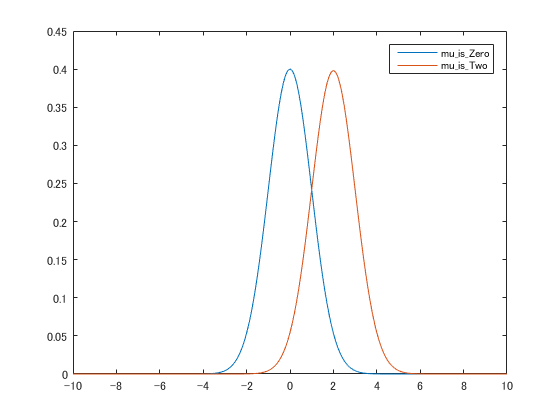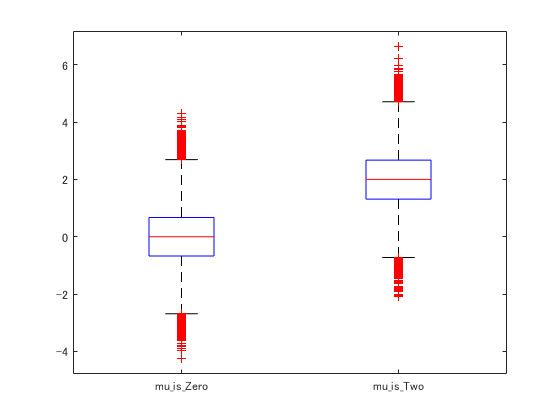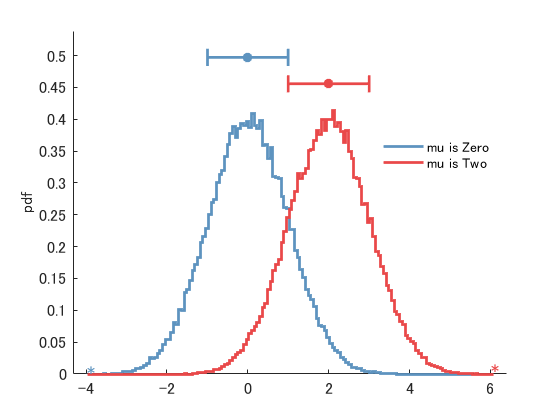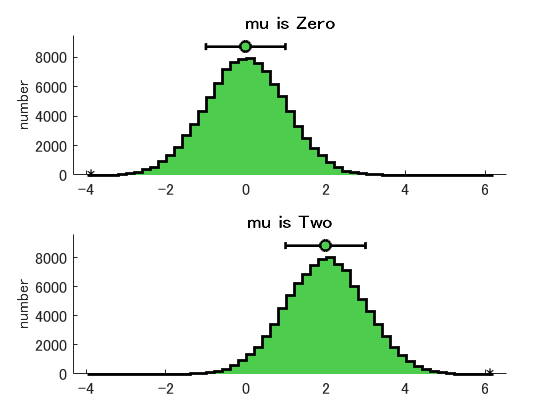# Comparing multiple histograms

Jiro's pick this week is "Comparing Multiple Histograms" by Jonathan C. Lansey.

One of the things you may want to do when analyzing two sets of data is comparing their distributions. There are various ways to do this. One is to fit each data set to a particular distribution using the function fistdist from the Statistics and Machine Learning Toolbox.

A.mu_is_Zero = randn(10^5,1);   % mean of 0
A.mu_is_Two = randn(10^5,1)+2;  % mean of 2

% This assumes you know the distribution
dist1 = fitdist(A.mu_is_Zero,'Normal')  % fit to a normal distribution
dist2 = fitdist(A.mu_is_Two,'Normal')   % fit to a normal distribution

x = -10:0.1:10;
y1 = pdf(dist1,x);
y2 = pdf(dist2,x);

plot(x,y1,x,y2)

legend(fieldnames(A),'interpreter','none')

dist1 =
NormalDistribution

Normal Distribution
mu = 0.00281815   [-0.00337045, 0.00900676]
sigma =    0.99848   [0.994123, 1.00288]

dist2 =
NormalDistribution

Normal Distribution
mu = 2.00247   [1.99627, 2.00868]
sigma = 1.00081   [0.996446, 1.00522]You can also use boxplot, also from Statistics and Machine Learning Toobox, to create a box and whisker plot that lets you visualize statistical information.

clf
boxplot([A.mu_is_Zero, A.mu_is_Two],'labels',fieldnames(A))Jonathan's nhist lets you compare the histograms of the data sets easily.

clf
nhist(A)

ans =
mu_is_Zero: 'mu_is_Zero: mean=0.00, std=1.00, 3 points counted in the ...'
mu_is_Two: 'mu_is_Two: mean=2.00, std=1.00, 1 points counted in the r...'Note that it automatically uses the field names for the legend.

Of course, this is just the default behavior. This function comes with a wealth of options for controlling everything from line properties and graph orientations to histogram properties and statistics displayed (mode, median, standard error, etc.). For example, to create two separate histograms with a greenish color and same number of bins,

nhist(A,'color',[.3 .8 .3],'separate','samebins','maxbins',50)

ans =
mu_is_Zero: 'mu_is_Zero: mean=0.00, std=1.00, 3 points counted in the ...'
mu_is_Two: 'mu_is_Two: mean=2.00, std=1.00, 1 points counted in the r...'Comments

There are many other options and the function comes with a very detailed help. Give it a try, and let us know what you think here or leave a comment for Jonathan.

Published with MATLAB® R2015b

|

### Comments

To leave a comment, please click here to sign in to your MathWorks Account or create a new one.